## Audio

[Audio][slideshow]

### Integrator circuit.

An integrator circuit is a circuit in which the input waveform. An integrator circuit based on opamp is shown in fig1. Such a circuit is also termed as an integrating amplifier. The circuit is somewhat similar to an opamp inverting amplifier but the feedback resistor Rf is replaced by a capacitor Cf. The circuit diagram of an opamp as an integrator is shown below.

###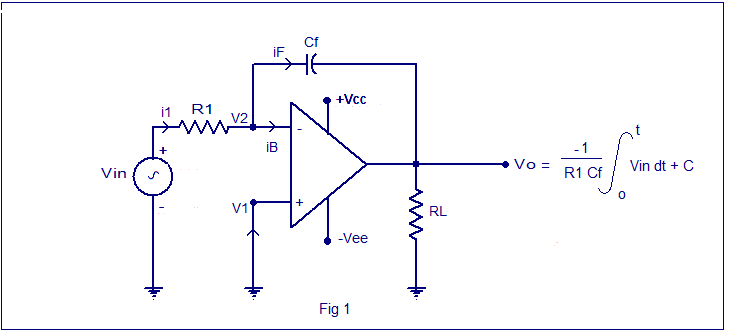Equation for the instantaneous output voltage of the opamp integrator can be derived as follows.

Applying Kirchoff’s current (KCL) at node V2 we get

i1= iF + iB

Since the input resistance of an opamp is very high (in the range of Mega ohms) iB will be very small at it can be neglected.

Therefore i1 = iF

The relation between the current through a capacitor and voltage across it is iC = C dv/dt.

Therefore iF = Cf X d (V2 – Vo ) / dt

Also i1 = (Vin – V2) / R1.

Therefore the equation i1 = iF can be rewritten as (Vin – V2) / R1 = Cf X d(V2 – Vo) / dt……….(1)

Since the non-inverting input is connected to ground, V1 can be taken as 0. Since the open loop gain of the present circuit is near infinity V2 can be assumed to be zero.

So the equation (1) becomes; Vin / R1 = Cf X d (-Vo) / dt

Integrating the both sides of the above equation with respect to time, we get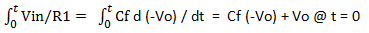Rearranging the equation we get :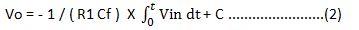C is the integration constant and it has a proportional relationship with the output voltage at time t = 0.From equation (2) it is clear that the output voltage has an inverse relation with the R1 Cf (time constant) and a directly proportional relation with the negative integral of the input voltage.

In the DC condition Cf offers infinite resistance and so the integrator circuit will be like an inverting opamp amplifier with infinite feedback resistance (Rf = ∞). The equation for the voltage gain (A) of an opamp amplifier in inverting mode is A = -(Rf/R1). Substituting Rf=∞ in the present scenario we get A=∞. Therefore the small input offset voltage will get amplified by this factor and there will be an error voltage at the output. This problem can be solved by adding a feedback resistor Rf parallel to Cf as shown in fig 4 shown below.Practical opamp integrator circuit

The addition of Rf will fix the low frequency gain (A) of the circuit to a fixed small value and so the input offset voltage will have practically no effect on the output offset voltage and variations in the output voltage is prevented.

Integrating a square wave will result in a triangle waveform and integrating a sine wave will result in a Cosine waveform. It is shown in the figures shown below.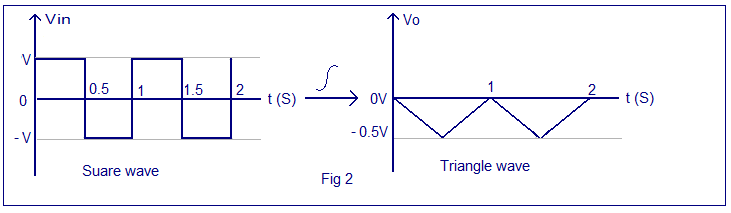Integrating square wave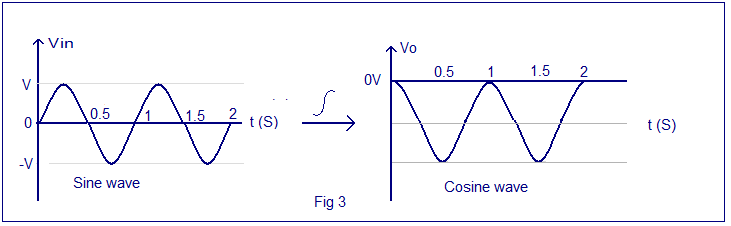Integrating sine wave waveform

[Lights][grids]